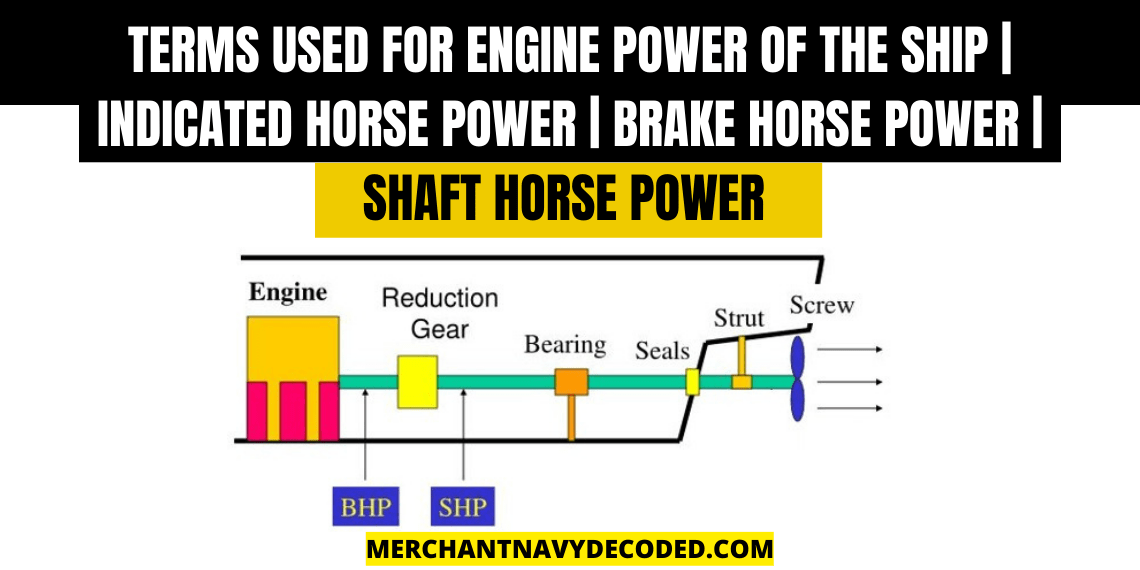## Terms used for engine power of the ship | Indicated Horse Power | Brake Horse Power | Shaft Horse Power

Last updated on May 20th, 2023 at 05:34 am

## What are the different methods of representing a marine engine’s performance?

### Indicated Horsepower(IHP)

It is the sum of the power generated by each cylinder inside the engine. Indicated horsepower is calculated per unit by the use of power card using the formula Pm x L x A x n, where Pm is the mean indicated pressure and n is the number of revolutions per second.

### Brake Horsepower(BHP)

BHP is the actual power output after the crankshaft. So, the power consumed in rotation of camshaft, rotation of flywheel, overcoming friction in bearings and other mechanical losses. In simple terms:

(BHP÷IHP)x100 = Mechanical Efficiency

Also onboard ship BHP is calculated by the formula BHP=Pe x L x A x n, where Pe is mean effective pressure and this mean effective pressure is generally equal to:

Pe=Pm-1

where Pm is mean indicated pressure

### Shaft Horsepower(SHP)

It is the amount of power which reaches the propeller after transmission losses through the shaft. The transmission losses occur because there are bearings needed to support and lubricate the shaft. Generally, this bearing is called intermediate shaft bearing.

SHP=0.99 x BHP

### Thrust Horsepower

It is the power that the propeller is able to produce. This is less than the shaft horsepower because of propeller losses, eddy losses and losses due to the mechanical considerations of the propeller.

THP/SHP = Propeller Efficiency

IHP>BHP>SHP>THP